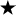home | O'Reilly's CD bookshelfs | FreeBSD | Linux | Cisco | Cisco ExamChapter 2Simple Graphics2.3 Dimension

The Dimension class is similar to the Point class, except it encapsulates a width and height in a single object. Like Point, Dimension is somewhat underused; it is used primarily by methods that need to return a width and a height as a single object; for example, getSize() returns a Dimension object.

Dimension Methods

Variables

A Dimension instance has two variables, one for width and one for height. They are accessible directly or through use of the getSize() method.

public int width

The width variable represents the size of an object along the x axis (left to right). Width should not be negative; however, there is nothing within the class to prevent this from happening.

public int height

The height variable represents the size of an object along the y axis (top to bottom). Height should not be negative; however, there is nothing within the class to prevent this from happening.

Constructors

public Dimension ()

This constructor creates a Dimension instance with a width and height of 0.

public Dimension (Dimension dim)

This constructor creates a copy of dim. The initial width is dim.width. The initial height is dim.height.

public Dimension (int width, int height)

This constructor creates a Dimension with an initial width of width and an initial height of height.

Sizing

public Dimension getSize ()The getSize() method retrieves the current size as a new Dimension, even though the instance variables are public.

public void setSize (int width, int height)The setSize() method changes the dimension's size to width x height.

public void setSize (Dimension d)The setSize() method changes the dimension's size to d.width x d.height.

Miscellaneous methods

public boolean equals (Object object)

The equals() method overrides the Object.equals() method to define equality for dimensions. Two Dimension objects are equal if their width and height values are equal.

public String toString ()

The toString() method of Dimension returns a string showing the current width and height settings. For example:

java.awt.Dimension[width=0,height=0]